## 6.12Mixing length

By analogy with kinetic theory, the eddy viscosity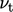can be expressed in terms of a characteristic speed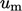and length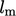. Prandtl produced the following model for the mixing length:16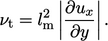(6.21)
The mixing length Eq. (6.21 ) is derived from the kinetic view of viscosity in Sec. 6.10 . Turbulent ﬂuctuations in the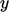-direction,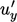, carry ﬂuid to the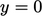plane from a distance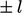.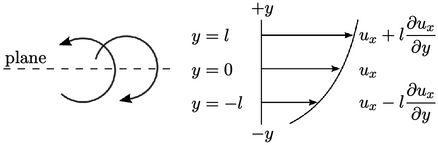At, the turbulent ﬂuctuations in the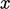-direction,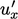, correspond to the range of velocities at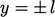such that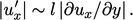For a positive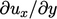, a positive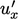at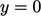corresponds to a negative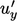, and vice versa. The Reynolds stress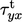can then be constructed by deﬁning a mixing length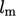to replace, absorbing the constant of proportionality, to give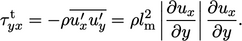(6.22)
From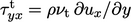, we arrive at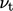in Eq. (6.21 ).

The mixing length Eq. (6.21) is only eﬀective as a turbulence model to calculate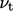when the ﬂow is simple enough that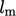can be chosen appropriately.

Such an example is high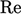, fully-developed ﬂow through a pipe of radius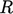. The mixing length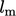, calculated from measured velocity proﬁles, follows a polynomial function of distance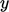from the wall, given by17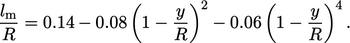(6.23)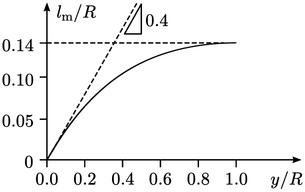Notably, close to the wall, e.g.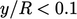, the mixing length increases linearly according to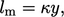(6.24)
where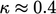is Kármán’s constant.

At the centre of the pipe,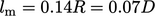where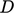is pipe diameter. Estimates of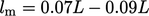are commonly cited for other simple examples, e.g. mixing layer, jet, ﬂat plate boundary layer, etc., where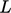is the characteristic length of the problem (radius in the case of a jet).

16Ludwig Prandtl, Bericht uber Untersuchungen zur ausgebildeten Turbulenz, 1925.
17Johann Nikuradse, Strömungsgesetze in rauhen Rohren, 1933.

Notes on CFD: General Principles - 6.12 Mixing length